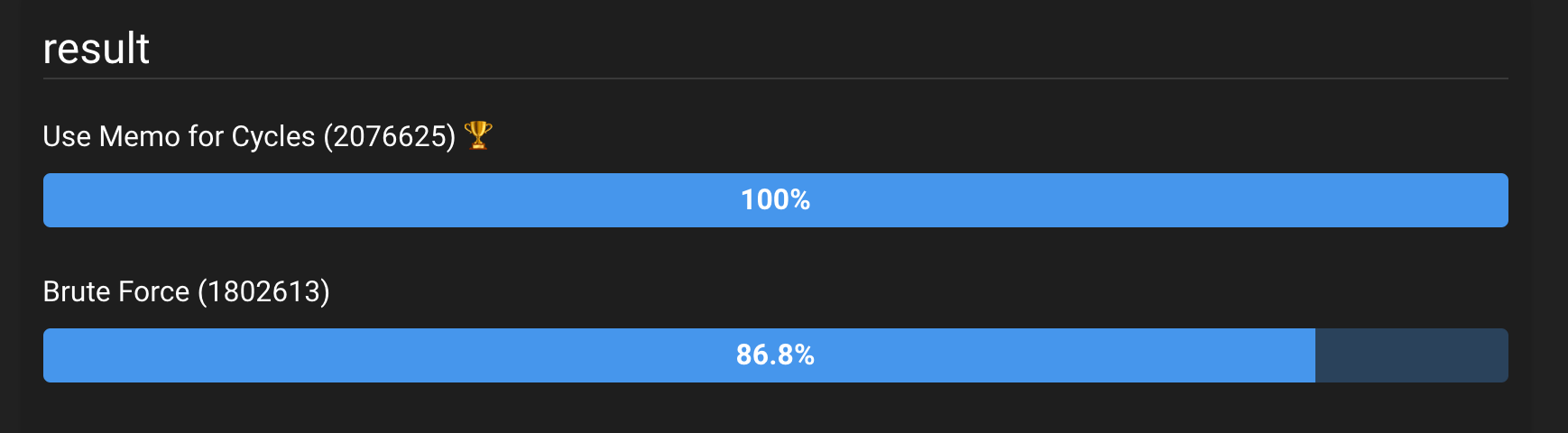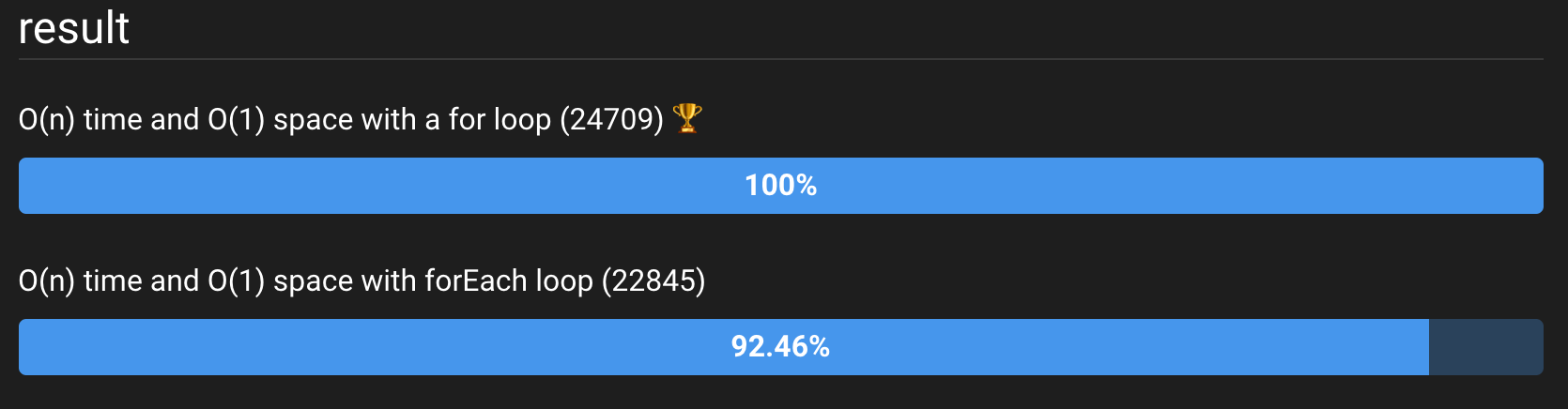# Deep dive into an interview question: Array Nesting

The Array Nesting interview question might seem complex at first and very simple after you solve it. But sometimes, a deeper dive into it can expose some more performance optimizations that can be interesting. After this article, you’ll be able to amaze your interviewers with some new insights they didn’t think of!

## What is the Array Nesting problem?

Let’s get into the problem itself.

Your input is an array `arr` of integers of length n. The integers themselves are some scrambling of the indices. So building the input might look like this:

For every member of the scrambled array, you should calculate a series that looks like this:

`s[k] = [arr[k], arr[arr[k]], arr[arr[arr[k]], ...]`

The stop rule is when you get to a number that you already visited, and do not include it.

Eventually, you should return the longest series in `s`.

### Example of a Array Nesting

Let’s take the following input: `[6, 5, 7, 8, 0, 4, 3, 2, 1, 9]`.

The series that are created from this array are:

``````  [3, 8, 1, 5, 4, 0, 6],
[4, 0, 6, 3, 8, 1, 5],
[2, 7],
[1, 5, 4, 0, 6, 3, 8],
[6, 3, 8, 1, 5, 4, 0],
[0, 6, 3, 8, 1, 5, 4],
[8, 1, 5, 4, 0, 6, 3],
[7, 2],
[5, 4, 0, 6, 3, 8, 1],
``````

From here it’s easy to see the longest series’ length is 7.

If you’ve never solved the Array Nesting problem, I suggest you solve the the problem first because solution spoilers are ahead. It can be found on leetcode.

If you’re more interested in the juicy part, just read on.

## How to solve the Array Nesting problem in an interview?

When solving a problem in an interview, you’d usually follow this pattern:

1. Solve a brute force solution

### Brute Force

The algorithm for our solution is pretty straight forward:

``````function getNestedArraysMaxLength(inputArray) {
let maxLength = -Infinity;

inputArray.forEach(value => {
const seriesLength = findSeriesLength(inputArray, value);
maxLength = Math.max(seriesLength, maxLength);
});

return maxLength;
}``````

We do exactly as we’ve been asked. For every member in `inputArray` we get its `seriesLength` and keep the maximum length found. We eventually return the maximum length as requested.

Now we just need to implement `findSeriesLength`:

``````function findSeriesLength(arr, nextValue) {
const series = {[nextValue]: true};

while (true) {
nextValue = arr[nextValue];
if (series[nextValue]) {
return Object.keys(series).length;
}
series[nextValue] = true;
}
}``````

This is the more complex bit of the algorithm. It gets the array and the next value (which is the first value in the series). The series always includes the first number, so we set it up in the series. We then fill the series until we get to the same number again. Once we do that, we return the length of the series.

The complexity of the Brute Force solution is O(n*n) (O n squared). That’s because we could, in the worse case, run on all the numbers in the original array and for each, run on them again when we compose the series. Usually, in interviews, we can do better.

### Optimize for time

When we look at the example shown above, we can detect a pattern:Figure 1: The pattern in the numbers. This illustration demonstrates the cyclic nature of our series. The first one starts from three and goes all the way to 6 (white arrows). The next one starts from 6, then 3 and goes the same way to 0 after which always comes 6 (blue arrow) and so on.

Figure 1 illustrates the cyclic nature of the series. The series are just cycles of the same numbers. We can use that in order to optimize our algorithm. Let’s add a memory array to keep counts of values we’ve been to before:

Let’s list the difference:

Line 3 defines the memory array.

Line 6 adds the count to the memory array instead of keeping it in a variable. We’re now using this value in line 7 instead of the `seriesLength` variable.

`findSeriesLength` now uses the memory it receives as input in order to check if we already have the count for a certain value (lines 18 to 20).

Let’s look at the complexity (big O) of this solution. We still go over the `n` values in the `inputArray`. But for each cycle as illustrated in figure 1, we will go only once. Our time complexity is now O(n) (linear) because the only place we go over `n` elements is in the main `forEach` loop. Our space complexity is now O(n) because we are now using the auxiliary `memory` array.

### Benchmarking

Let’s see the difference between the optimized and non optimized algorithms. We will use the website jsben.ch for our performance benchmarking. Our benchmarking will start with n = 10 (yes, I know it’s small, but bear with me please).Figure 2: The results of running the two solutions a lot of times. You can see that the solution using the memo for cycles was around 15% better.

Figure 2 (you can see the live benchmark here: https://jsben.ch/GQld2) shows that the optimization worked. Although… we’d expect a much better improvement, no? We improved our complexity by a lot!

## Can we optimize more?

There are several ways to optimize our algorithm. For starters, let’s see what happens when we use a counter instead of the `series` array inside the `findSeriesLength` function. We will change the function to be like this:

``````function findSeriesLength(arr, nextValue) {
const startingNumber = nextValue;
let count = 1;

while (true) {
nextValue = arr[nextValue];

if (startingNumber === nextValue) {
return count;
}
count++;
}
}``````

In the code above, instead of creating the series, which is not needed for our task (we need only return the length), we remember the first value (`startingNumber`) and create a counter.

The benchmark for this function is shown in Figure 3 (live version here).Figure 3: Results of benchmarking Brute Force with the counter (Brute Force less GC) against the Brute Force and the optimized algorithm (Use Memo for Cycles). This resulted in a huge garbage collection reduction which made the brute force O(n*n) even more efficient than the O(n) algorithm.

What would the same optimization do to the complexity optimization? The results shown in Figure 4 might surprise you.Figure 4: Results of the O(n) vs O(n*n) with the optimized findSeriesLength function. Oh and behold – the O(n) algorithm is running slower than the Brute Force!!! Live code

What’s going on here? Can a brute force O(n*n) algorithm run faster than an O(n) algorithm?

Garbage Collection (GC in short) is a major factor in JavaScript performance. If a function creates and destroys a lot of objects/arrays, it will incur a price: memory allocation and garbage collection. The JS engine has to allocate memory for your Objects and Arrays. The JS engines also clears discarded objects and arrays (the garbage collection). These processes take time. At scale – it takes even longer.

In addition, the O(n) algorithm, as shown here, is not giving the benefits we’d expect from a much more efficient algorithgm. That’s because we have a very low `n`. If we increase `n` to 1000, we will see a different picture. Figure 5 shows the results for `n = 1000`.Figure 5: The same code, but this time the input array is of length 1000. Watch the live demo here: https://jsben.ch/1EWkg

So we learn 2 things here:

1. Complexity improvements are good for bigger `n`‘s.
2. GC can be a major bottleneck in your JavaScript code.

### Optimize for time and space

We can also improve the time complexity without increasing the space complexity and completely avoid the GC overhead.

Instead of creating the memory array, we can just set the marker inside our array:

Note that we removed any mention of `memory`. The trick here is to set every value we already pass as null (or some other known value). Then, every time we stumble upon `null` we know we’ve already checked this cycle, and there’s no need to go farther.

The results in Figure 6 are quite decisive. Using O(n) time and improving to O(1) in space also resulted in better performance. We already know the reason for that – memory allocation and garbage collection. When we do not allocate the `memory` array, we actually reduce the amount of memory allocated and thus reduce the amount of GC cycles.Figure 6: Comparing all algorithms so far to the improvement of O(n) time and O(1) in space. See the live tests here: https://jsben.ch/zRThi

In order to prove our hypothesis, we can easily remove the GC from the O(n) space solution. By changing the line `const memory = [];` to `const memory = new Array(inputArray.length).fill(1);` we will preallocate the memory before the function starts and thus have a lot less GC cycles and memory allocation requests. See the results in Figure 7.Figure 7: Results for the O(n) optimizations – dynamically allocating memory (bottom) takes significantly longer time to run than preallocated memory (middle). Preallocated memory takes about the same amount of time to run as the fully optimized code. Live code here: https://jsben.ch/Gllfr

## Is there anything else?

Of course! But from now on, it’s probably going to be micro optimization that depends on the browser you are using or the JS engine you are using. For instance, if we change the `forEach` with a `for` loop, we’ll see the following improevement:Figure 8: Results of micro optimization – replacing the forEach with a for loop. We see a small increase in performance. This might vary with browser and JS engine. See the live code here: https://jsben.ch/CRdBH

## Summary

The Array Nesting task is a relatively simple interview problem. Its brute force solution is quite straight forward and its optimizations are simple: either save a memory of old results (memoization) or use a flag on the data itself.

We also saw that the way we handle the data can affect the solution’s speed. Memory allocation and Garbage Collection have a high impact on your code’s performance.

Finally, we saw that some optimizations that achieved popularity due to their “fun fact” and rather notorious nature (like replacing the forEach) are less likely to give you better performance.

For our task – retrieving the longest series – our data handling was optimized as we did. We either kept a counter or we pre allocated the `memory` array.

I hope that now, if asked this question in an interview, you’ll be able to woo your interviewer with deep explanations about memory allocation and garbage collection, after you finish explaining the obvious big O complexity.

You can read more about memory allocation and garbage collection and how to detect and solve them in your code.

If you didn’t so far, you can try to rest a while and then try to solve the challenge on leetcode.

Thanks to Miki Ezra Stanger for the very kind and helpful review.

Featured Photo by Marty Southwell on Unsplash